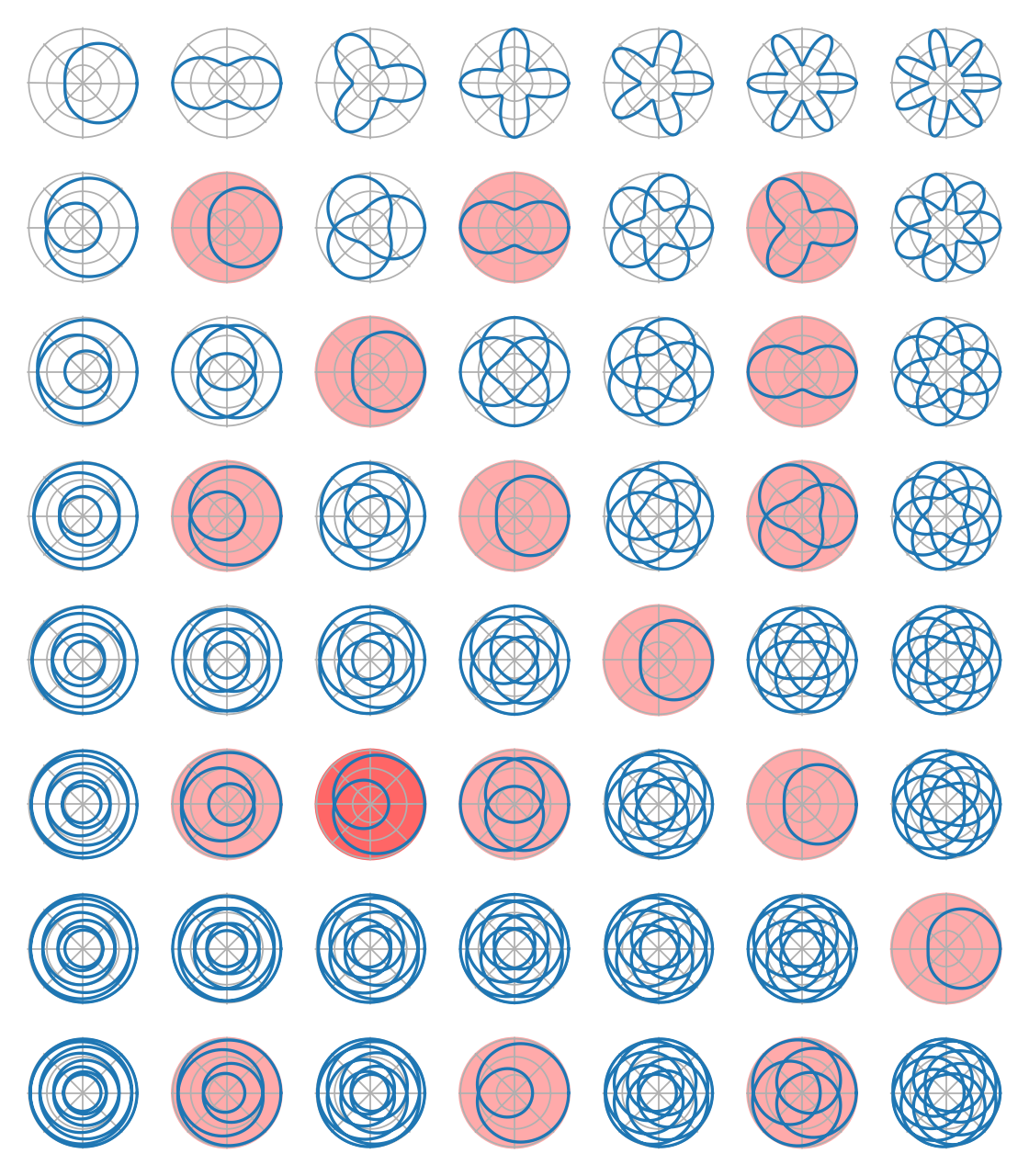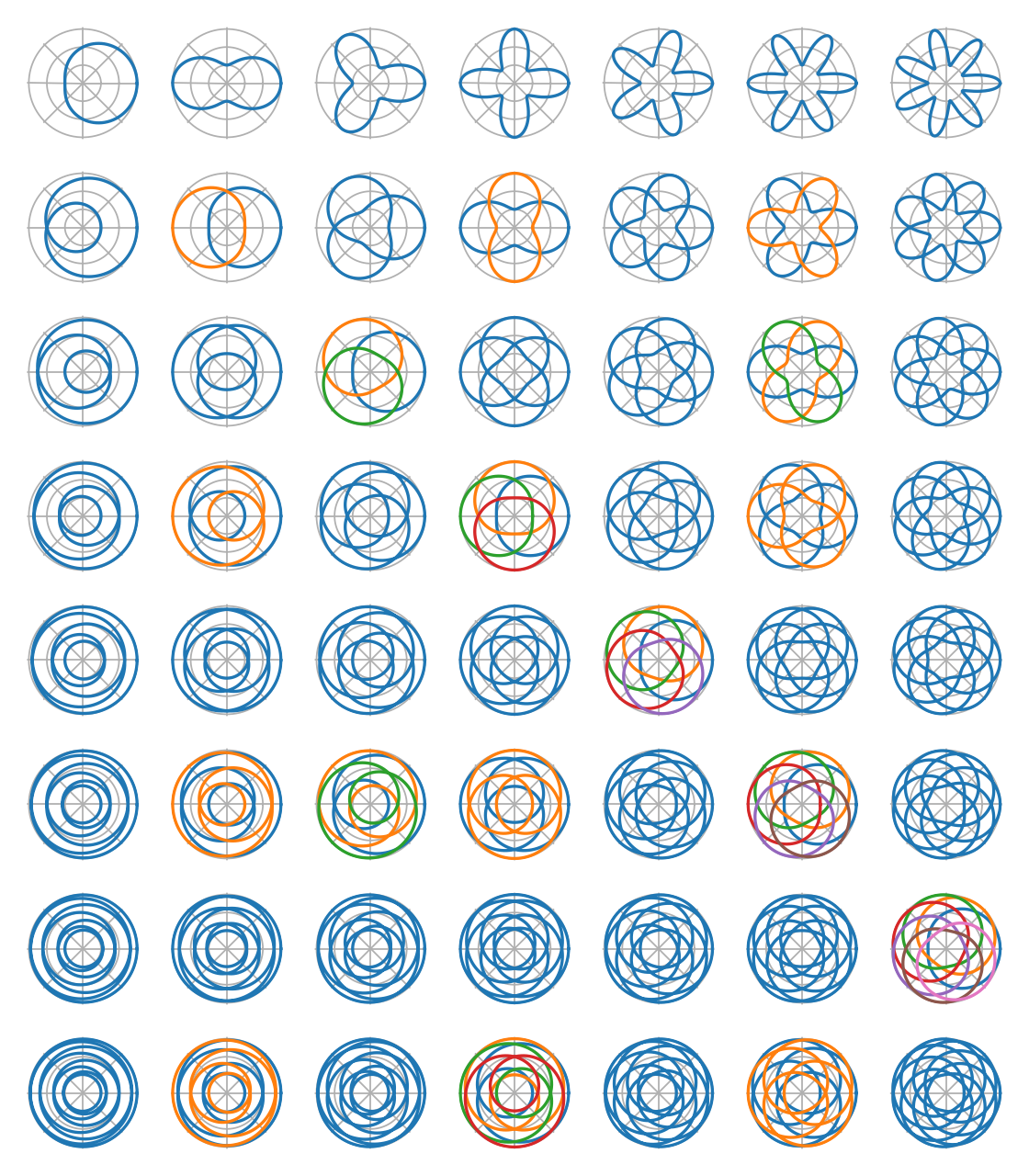# Intuition¶

Let’s find a family of curves looking like Turk’s head knots.

## General shape¶

Looking at the flat form of Turk’s head knots, we see that the string oscillates between the center and the outside while it turns around the center. This is why the first idea is to draw some sine wave is polar coordinates. (Oscillation means sine wave, turning around the center means polar coordinates)

Since we don’t want the curve to go through the center of the image, we have to keep the radius strictly positive, so we will draw $$r = r_0+\delta_r \cdot \cos(\alpha \cdot \theta)$$, with $$0 < \delta_r < r_0$$.

The next question is how to fix $$\alpha$$. For a knot with $$q$$ leads, the string makes $$q$$ turns around the center, so we will draw the wave for $$\theta \in [0, 2 \cdot q \cdot \pi]$$. Then, for a knot with $$p$$ bights, the string touches the center $$p$$ times, and the outside $$p$$ times. Thus, the wave must have $$p$$ maxima and $$p$$ minima on the range. $$\cos$$ has exactly one maximum and one minimum on $$[0, 2 \cdot \pi]$$, so we can use $$\alpha=p/q$$. This gives us:

\begin{align}\begin{aligned}r = r_0 + \delta_r \cdot \cos \left(\frac{p \cdot \theta}{q} \right)\\\theta \in \left[0, 2 \cdot q \cdot \pi \right[\end{aligned}\end{align}

Let’s draw this with $$r_0 = 2$$ and $$\delta_r = 1$$ for for small values of $$p$$ and $$q$$:

import numpy as np
import matplotlib.pyplot as plt
import fractions

P = 7
Q = 8

plt.figure(figsize=(P, Q))

for p in range(1, P + 1):
for q in range(1, Q + 1):
d = fractions.gcd(p, q)  # Explained below

r = lambda theta: 2 + np.cos(p * theta / q)
theta = np.arange(0, 2 * q * np.pi / d, 0.01)

if d == 1:
bg = "white"
elif (p, q) == (3, 6):
bg = "#ff6666"
else:
bg = "#ffaaaa"
sp = plt.subplot(Q, P, (q - 1) * P + p, polar=True, facecolor=bg)
sp.plot(theta, r(theta))
sp.set_rmin(0)
sp.set_rmax(3.1)
sp.set_yticks([1, 2, 3])
sp.set_yticklabels([])
sp.set_xticklabels([])
sp.spines['polar'].set_visible(False)

plt.tight_layout()In the previous figure, $$q = 1$$ on the first line (the string makes one turn around the center) and $$p = 1$$ on the left column (the string touches the outside once).

## Adjustment for several strings¶

This is mainly ok, but cases on red background look wrong (For example, $$p = 3$$ and $$q = 6$$, with a darker red background). This is due to the known result that, if $$d = \gcd(p, q)$$, you need $$d$$ strings to make a Turk’s head knot with $$p$$ bights and $$q$$ leads. This means that we have to draw $$d$$ curves. The total number of turns around the center is still the same, so each string makes $$q/d$$ turns, and the range for $$\theta$$ is reduced to $$[0, 2 \cdot q \cdot \pi / d]$$ for each curve.

Since the second curve must draw the second bight, it means that the second curve must be shifted by $$2 \cdot \pi / p$$. Extending this result tells us that the $$k^{th}$$ curve must be shifted by $$2 \cdot k \cdot \pi / p$$. So $$r_k = r_0 + \delta_r \cdot \cos \left(\frac{p \cdot (\theta - 2 \cdot k \cdot \pi / p)}{q} \right)$$.

This give use our final family of curves:

\begin{align}\begin{aligned}r_k = r_0 + \delta_r \cdot \cos \left( \frac{p \cdot \theta - 2 \cdot k \cdot \pi}{q} \right)\\\theta \in \left[0, \frac{2 \cdot q \cdot \pi}{d} \right[\\k \in [0, d - 1]\end{aligned}\end{align}
import numpy as np
import matplotlib.pyplot as plt
import fractions

P = 7
Q = 8

plt.figure(figsize=(P, Q))

for p in range(1, P + 1):
for q in range(1, Q + 1):
d = fractions.gcd(p, q)

r = []
for k in range(d):
r.append(
lambda theta, k=k: 2 + np.cos((p * theta - 2 * k * np.pi) / q)
)
theta = np.arange(0, 2 * q * np.pi / d, 0.01)

sp = plt.subplot(Q, P, (q - 1) * P + p, polar=True)
for k in range(d):
sp.plot(theta, r[k](theta))
sp.set_rmin(0)
sp.set_rmax(3.1)
sp.set_yticks([1, 2, 3])
sp.set_yticklabels([])
sp.set_xticklabels([])
sp.spines['polar'].set_visible(False)

plt.tight_layout()## Ups and downs¶

In a Turk’s head knot, each string alternate between going up and going down every time it crosses itself or another string. So we’ll need to compute intersection points between curves of our family. Then we’ll assign alternative “altitudes” to the curves at those points. A simple linear interpolation will give us the altitude of the string between two known altitudes. This could be made smoother if we wanted to build a 3D model of the knot, but this is enough to draw it from above.